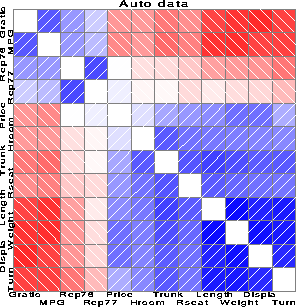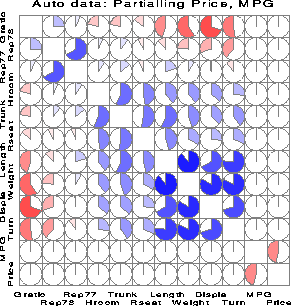corrgram Draw a correlogram corrgram

# SAS Macro Programs: corrgram

\$Version: 1.2-3 (21 Sep 2012)
Michael Friendly
York University

## The corrgram macro (get corrgram.sas)

### Draw a correlogram

The CORRGRAM macro produces a schematic display of a correlation matrix, called a correlogram. In this display, variables are permuted so that ``similar'' variables are positioned adjacently, and cells of a matrix are shaded or filled to show the correlation value.

## Usage

The CORRGRAM macro is defined with keyword parameters. The arguments may be listed within parentheses in any order, separated by commas. For example:
```  %corrgram(data=baseball, var=_numeric_, fill=C E C);
```
The input dataset may contain raw data (no missing values, yet), or a correlation matrix (type='CORR') produced by PROC CORR or some other SAS procedure.

### Parameters

Default values are shown after the name of each parameter.
DATA=
Name of input data set. [Default: `DATA=_LAST_`]
VAR=
List of variables whose correlations are to be displayed. You may use an explicit, blank separated list of variables (the case of variable names will be preserved), or any of the SAS abbreviations, such as `X1-X15`, `height--strength`, or `_NUMERIC_` (variable labels will apprear in upper case).
TYPE=
Type of input data: DATA or CORR. If not specified, the program tries to guess, by examining the type attribute of the input data set.
FILL=
How to fill the cells in the lower triangle, diagonal and the upper triangle. Should be three characters, chosen from `S=` shade (with color proportional to the correlation), `E=` empty, `N=` numeric value, `C=` circle, or `B=` bar [Default: `FILL=S E S`]
ORDERBY=
PCs used to order the variables, either one or two integers (eigenvector numbers) or empty (ORDERBY=). [Default: `ORDERBY=1 2`]
OPTIONS=
One or more options: RINV displays R-1 rather than R. TRANS transposes the data set, and calculates correlations among observations, rather than variables.
PARTIAL=
List of one or more variables to be partialed out. These variables must be included in the VAR= list.
COLORS=
Colors for positive and negative values [Default: `COLORS=BLUE RED`]
HLABEL=
Height of variable labels
ALABEL=
Angles for variable labels on the vertical and horizontal axes.
CMIN=
Minimum absolute value for correlation to be shaded.
TITLE=
Optional plot title, or `NONE`, for no title. Under V7+, the macro uses the `TITLE1` unless `TITLE=NONE` has been specified.

### Example

These examples display correlograms for correlations among variables on automobiles.
```goptions hsize=6in vsize=6in;     *-- make plot square;

%include data(auto);
data auto;
set auto;
if rep77 ^= . and rep78 ^=.;       /* delete missing data         */

%include macros(corrgram);        *-- or include in an autocall library;

%corrgram(data=auto,
var = Gratio  Turn  Rep77  Rep78  Price    MPG
Hroom Rseat  Trunk Weight Length Displa,
orderby=2 1,
title=Auto data);
```Here, we calculate and display partial correlations, partialling Price and MPG. The values are represented by circular 'pac-man' symbols, using the option FILL=C E C.

```%corrgram(
var = Gratio  Turn  Rep77  Rep78  Price    MPG
Hroom Rseat  Trunk Weight Length Displa,
orderby=2 1,
partial=price mpg ,
fill = C E C,
/* alabel=-25 65, */
title=%str(Auto data: Partialling Price, MPG));
```### See also

biplot Biplot display of variables and observations
canplot Canonical discriminant structure plot
faces Faces display for multivariate data